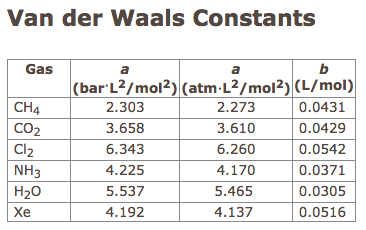# Problem: Use the van der Waals equation of state to calculate the pressure of 2.70 mol of Xe at 473 K in a 5.50-L vessel. Van der Waals constants can be found below.P= ______________ atmUse the ideal gas equation to calculate the pressure under the same conditions.P=_______________atm

###### FREE Expert Solution
95% (277 ratings)
###### Problem Details

Use the van der Waals equation of state to calculate the pressure of 2.70 mol of Xe at 473 K in a 5.50-L vessel. Van der Waals constants can be found below.

P= ______________ atm

Use the ideal gas equation to calculate the pressure under the same conditions.

P=_______________atmFrequently Asked Questions

What scientific concept do you need to know in order to solve this problem?

Our tutors have indicated that to solve this problem you will need to apply the Van der Waals Equation concept. You can view video lessons to learn Van der Waals Equation. Or if you need more Van der Waals Equation practice, you can also practice Van der Waals Equation practice problems.

What is the difficulty of this problem?

Our tutors rated the difficulty ofUse the van der Waals equation of state to calculate the pre...as high difficulty.

How long does this problem take to solve?

Our expert Chemistry tutor, Dasha took 9 minutes and 29 seconds to solve this problem. You can follow their steps in the video explanation above.

What professor is this problem relevant for?

Based on our data, we think this problem is relevant for Professor Johnson's class at UBC.